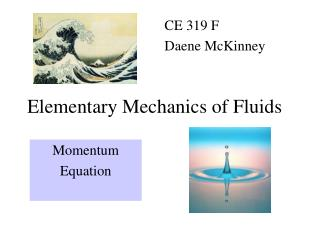Download PresentationElementary Mechanics of Fluids

# Elementary Mechanics of Fluids - PowerPoint PPT PresentationDownload Presentation## Elementary Mechanics of Fluids

- - - - - - - - - - - - - - - - - - - - - - - - - - - E N D - - - - - - - - - - - - - - - - - - - - - - - - - - -
##### Presentation Transcript

1. Elementary Mechanics of Fluids CE 319 F Daene McKinney Momentum Equation

2. Momentum Equation • Reynolds Transport Theorem • b = velocity; Bsys = system momentum • Vector equation -- 3 components, e.g., x

3. T=15 oC v=20 m/s d=30 mm Tank m=20 kgVo=20 L Ex (6.3) • Given: Figure • Find: (a) Force acting on bottom of the tank and (b) the force acting on the stop block. • Solution: W F N

4. W F N Ex (6.3)

5. T=15 oC F B Q=0.4 m3/s pA=75 kPa EX (6.5) • Given: Figure • Find: Horizontal force required to hold plate in position • Solution:

6. HW (6.12)

7. V1=28 m/s Q=0.20 m3/s V2=27 m/s Ex (6.17) Fy • Given: Figure • Find: External reactions in x and y directions needed to hold fixed vane. • Solution: Fx

8. V1=28 m/s Q=0.20 m3/s V2=27 m/s Ex (6.17) Fy Fx

9. y x Ex (6.34) D=30 cm Vol=0.10 m3 W=500 N • Given: Figure • Find: Force applied to flanges to hold pipe in place • Solution: Continuity equation • Momentum P=100 kPa, gage Q=0.60 m3/s p1A1 Fy V1 p2A2 Fx V2 Wb+Wf

10. HW (6.37)

11. Ex (6.72) Fy V2 • Given: Water jet, 6 cm diameter, with velocity 20 m/s hits vane moving at 7 m/s. • Find: Find force on vane by water. • Solution: Select CV moving with the vane at constant velocity. The magnitude of the velocity along the vane is constant Fx

12. HW (6.80)

13. HW (6.98)

14. HW (6.101)

15. Sluice Gate • Find: Force due to pressure on face of gate • Solution: Assume: v1 and v2 are uniform (so pressure is hydrostatic)